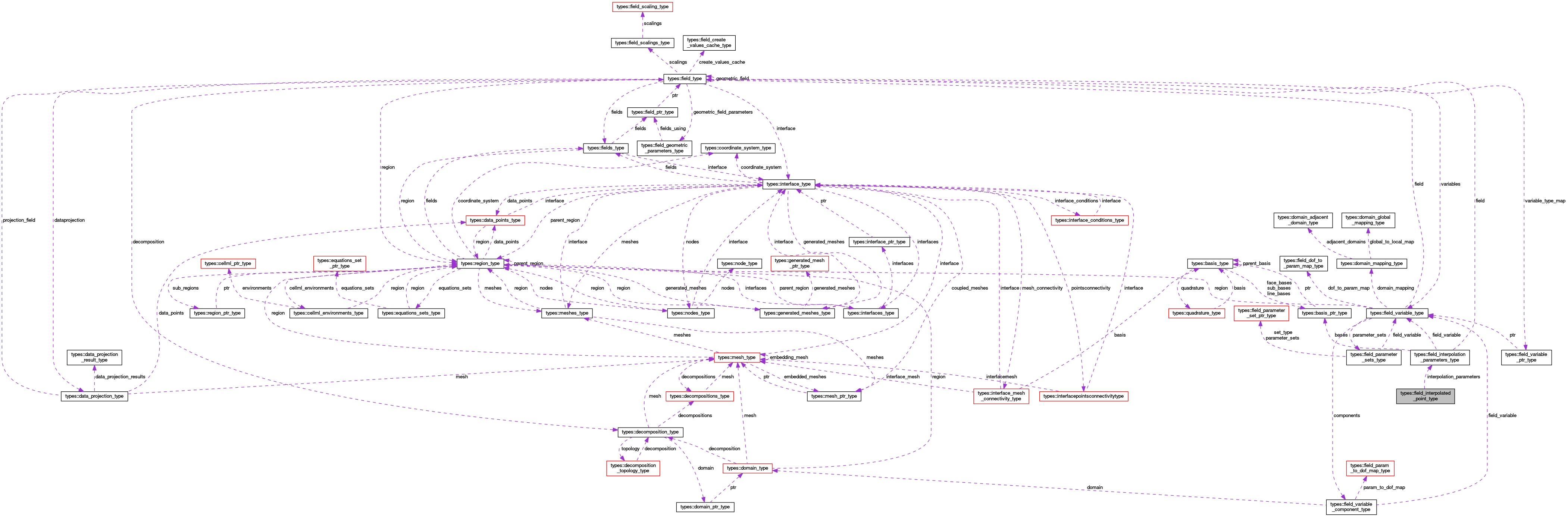OpenCMISS-Iron Internal API Documentation
types::field_interpolated_point_type Type Reference

Contains the interpolated value (and the derivatives wrt xi) of a field at a point. Old CMISS name XG. More...

Collaboration diagram for types::field_interpolated_point_type:[legend]

## Public Attributes

type(field_interpolation_parameters_type), pointer interpolation_parameters
A pointer to the interpolation parameters of the field that is to be interpolated. More...

integer(intg) max_partial_derivative_index
The maximum number of partial derivatives that have been allocated for the values component. More...

integer(intg) partial_derivative_type
The type of the partial derivatives that have been interpolated. PARTIAL_DERIVATIVE_TYPE can be either NO_PART_DERIV, FIRST_PART_DERIV or SECOND_PART_DERIV depending on whether just the field value, the field value and all first derivatives (including cross derivatives) or the first value and all first and second derivatives have been interpolated. More...

real(dp), dimension(:,:), allocatable values
VALUES(component_idx,nu). The interpolated field components and their partial derivatives. More...

## Detailed Description

Contains the interpolated value (and the derivatives wrt xi) of a field at a point. Old CMISS name XG.

Definition at line 1129 of file types.f90.

## Member Data Documentation

 type(field_interpolation_parameters_type), pointer types::field_interpolated_point_type::interpolation_parameters

A pointer to the interpolation parameters of the field that is to be interpolated.

Definition at line 1130 of file types.f90.

 integer(intg) types::field_interpolated_point_type::max_partial_derivative_index

The maximum number of partial derivatives that have been allocated for the values component.

Definition at line 1131 of file types.f90.

 integer(intg) types::field_interpolated_point_type::partial_derivative_type

The type of the partial derivatives that have been interpolated. PARTIAL_DERIVATIVE_TYPE can be either NO_PART_DERIV, FIRST_PART_DERIV or SECOND_PART_DERIV depending on whether just the field value, the field value and all first derivatives (including cross derivatives) or the first value and all first and second derivatives have been interpolated.

Definition at line 1132 of file types.f90.

 real(dp), dimension(:,:), allocatable types::field_interpolated_point_type::values

VALUES(component_idx,nu). The interpolated field components and their partial derivatives.

Definition at line 1133 of file types.f90.